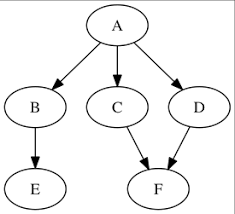# An Overview to the Workings of Graphs in Data Structures

### A quick introduction to graphs in data structures and how graphs can be used to depict the real-life relationships between different types of data.

· Big Data Zone · Tutorial
Save
5.87K Views

This article is aimed at providing a basic introduction of the graphs in data structures and the algorithms that are commonly used for traversing these graphs. Graphs and algorithms are the subjects some of the most frequently asked questions in the interview process.Graphs are one of the most interesting data structures in computer science, they are a powerful and versatile tool that lets us represent the relationship between various types of data easily. The two main parts of a graph are vertices (nodes) in which the data is stored, i.e., the letters in the image above and the edges that connect these nodes to each other.

Graphs can be undirected and directed.

• Undirected Graph: An undirected graph means that if we have an edge between two nodes, A and B, then we assume that there is a path from A to B as well as from B to A.
• Directed Graph: A directed graph has arrows on the edges which represent the direction of the relationship, i.e., if we have an edge between two nodes, A and B, directed from A to B, then the path exists from A to B only and not from B to A.
• Weighted Graphs: This type of graph has a weight or number associated with each edge.Now let us talk about the algorithms that are used for traversing the graphs. Traversing a graph means examining all the nodes and vertices of the graph. There are two general methods which are used for traversing a graph:

• Depth First Search: Depth First Search (DFS) starts with the initial node and then goes deeper and deeper until we find the goal node or the node which has no children. Once we reach a node that has no more children then we backtrack to the most recent node which still has nodes to explore. Stack is a data structure which uses the DFS algorithm.

## Steps for DFS:

Step 1: SET STATUS = 1 (ready state) for each node in G.

Step 2: Push the starting node A on the stack and set its STATUS = 2 (waiting state).

Step 3: Repeat Steps 4 and 5 until STACK is empty.

Step 4: Pop the top of node N. Process it and set its STATUS = 3 (processed state).

Step 5: Push on the stack all the neighbours of N that are in the ready state (whose STATUS = 1) and set their STATUS = 2 (waiting state)
[END OF LOOP]

Step 6: EXIT

For example:Traversing this graph using DFS, we’ll get:

A ---> B ---> E ---> C ---> F ---> D

• Breadth First Search: The Depth First Search (DFS) algorithm starts traversing the graph from the root node and moves on to exploring all its adjacent nodes. Once all its adjacent nodes are explored then it selects a neighboring node and explores all of its adjacent nodes. This process is repeated until we reach the goal node.

## Steps for BFS:

Step 1: SET STATUS = 1 (ready state) for each node in G

Step 2: Enqueue the starting node A and set its STATUS = 2 (waiting state)

Step 3: Repeat Steps 4 and 5 until QUEUE is empty

Step 4: Dequeue a node N. Process it and set its STATUS = 3 (processed state).

Step 5: Enqueue all the neighbors of N that are in the ready state (whose STATUS = 1) and set their STATUS = 2 (waiting state) [END OF LOOP]

Step 6: EXIT

For Example:Traversing this graph using BFS, we’ll get:

A ---> B ---> C ---> D ---> E ---> F

I hope this article helped in providing a quick introduction to graphs in data structures and how graphs can be used to depict the real-life relationships between different types of data.

Topics:
graphs, data structure, bfs, dfs, big data, tutorial

Opinions expressed by DZone contributors are their own.